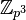# Direct product of cyclic group of prime-cube order and cyclic group of prime order

View other such prime-parametrized groups

## Definition

This group is defined as the external direct product of the cyclic group of prime-cube order (denoted$\mathbb{Z}_{p^3}$ or$C_{p^3}$) and the cyclic group of prime order (denoted$\mathbb{Z}_p$ or$C_p$), i.e., it is defined as$\mathbb{Z}_{p^3} \times \mathbb{Z}_p$ (or, in alternative notation,$C_{p^3} \times C_p$). It corresponds to the partition (see classification of finitely generated abelian groups and abelian group of prime power order):$\! 4 = 3 + 1$

For a given prime$p$, it can also be defined as the unique (up to isomorphism) abelian group of order$p^4$ and exponent$p^3$.

## Particular cases

Value of prime number$p$ Corresponding group
2 direct product of Z8 and Z2
3 direct product of Z27 and Z3
5 direct product of Z125 and Z5

## Arithmetic functions

Function Value Similar groups Explanation for function value
prime-base logarithm of order 4 groups with same prime-base logarithm of order
max-length of a group 4 max-length of a group equals prime-base logarithm of order for group of prime power order
chief length 4 chief length equals prime-base logarithm of order for group of prime power order
composition length 4 composition length equals prime-base logarithm of order for group of prime power order
prime-base logarithm of exponent 3 groups with same prime-base logarithm of order and prime-base logarithm of exponent | groups with same prime-base logarithm of exponent
Frattini length 3 groups with same prime-base logarithm of order and Frattini length | groups with same Frattini length Frattini length equals prime-base logarithm of exponent for abelian group of prime power order
minimum size of generating set 2 groups with same prime-base logarithm of order and minimum size of generating set | groups with same minimum size of generating set
subgroup rank of a group 2 groups with same prime-base logarithm of order and subgroup rank of a group | groups with same subgroup rank of a group same as minimum size of generating set since it is an abelian group of prime power order
rank of a p-group 2 groups with same prime-base logarithm of order and rank of a p-group | groups with same rank of a p-group same as minimum size of generating set since it is an abelian group of prime power order
normal rank of a p-group 2 groups with same prime-base logarithm of order and normal rank of a p-group | groups with same normal rank of a p-group same as minimum size of generating set since it is an abelian group of prime power order
characteristic rank of a p-group 2 groups with same prime-base logarithm of order and characteristic rank of a p-group | groups with same characteristic rank of a p-group same as minimum size of generating set since it is an abelian group of prime power order
nilpotency class 1 The group is a nontrivial abelian group
derived length 1 The group is a nontrivial abelian group
Fitting length 1 The group is a nontrivial abelian group

## GAP implementation

### Group ID

This finite group has order p^4 and has ID 5 among the group of order p^4 in GAP's SmallGroup library. It can thus be defined using GAP's SmallGroup function as:

SmallGroup(p^4,5)

For instance, we can use the following assignment in GAP to create the group and name it$G$:

gap> G := SmallGroup(p^4,5);

Conversely, to check whether a given group$G$ is in fact the group we want, we can use GAP's IdGroup function:

IdGroup(G) = [p^4,5]

or just do:

IdGroup(G)

to have GAP output the group ID, that we can then compare to what we want.